Hydrol. Earth Syst. Sci., 24, 6033–6046, 2020
https://doi.org/10.5194/hess-24-6033-2020
Hydrol. Earth Syst. Sci., 24, 6033–6046, 2020
https://doi.org/10.5194/hess-24-6033-2020

Technical note 22 Dec 2020

Technical note | 22 Dec 2020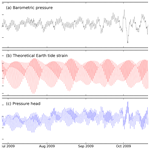# Technical note: Disentangling the groundwater response to Earth and atmospheric tides to improve subsurface characterisation

Technical note: Disentangling the groundwater response to Earth and atmospheric tides to improve subsurface characterisation
Gabriel C. Rau1,2, Mark O. Cuthbert3,2, R. Ian Acworth2, and Philipp Blum1 Gabriel C. Rau et al.
• 1Institute of Applied Geosciences (AGW), Karlsruhe Institute of Technology (KIT), Karlsruhe, Germany
• 2School of Civil and Environmental Engineering, The University of New South Wales (UNSW), Sydney, Australia
• 3School of Earth and Ocean Sciences, Cardiff University, Cardiff, United Kingdom

Correspondence: Gabriel C. Rau (gabriel.rau@kit.edu)

Abstract

The groundwater response to Earth tides and atmospheric pressure changes can be used to understand subsurface processes and estimate hydraulic and hydro-mechanical properties. We develop a generalised frequency domain approach to disentangle the impacts of Earth and atmospheric tides on groundwater level responses. By considering the complex harmonic properties of the signal, we improve upon a previous method for quantifying barometric efficiency (BE), while simultaneously assessing system confinement and estimating hydraulic conductivity and specific storage. We demonstrate and validate this novel approach using an example barometric and groundwater pressure record with strong Earth tide influences. Our method enables improved and rapid assessment of subsurface processes and properties using standard pressure measurements.

Share
1 Introduction

The groundwater response to barometric pressure and gravity changes caused by Earth tides has long been observed and is a powerful yet underutilised tool to passively characterise subsurface systems . While atmospheric pressure changes act as a load on the subsurface, and its groundwater pressure response can be related to compressible properties of the formation (e.g. Clark1967; Davis and Rasmussen1993), Earth tides cause areal strain, resulting in small pore pressure changes . The main research focus has long been the removal of both signals from the groundwater pressure in order to better understand and quantify processes such as pumping tests or recharge (e.g. Rojstaczer and Agnew1989). However, tidal forces are ubiquitous, and their groundwater response can therefore also be utilised to quantify in situ hydro-geomechanical properties . Tidal harmonic components have long been named depending on their frequency (Agnew2007). A comprehensive list of the most common components found in groundwater head measurements is summarised in Table 1 (Merritt2004). reviewed the state of the science, highlighted the potential for such passive approaches and coined the term tidal subsurface analysis (TSA).

Table 1Overview of the major tidal components found in well water levels grouped by mode (diurnal and semi-diurnal) and ordered by frequency. Columns BP, ET and GW show which component can occur in what type of record. Note: cpd – cycle per day.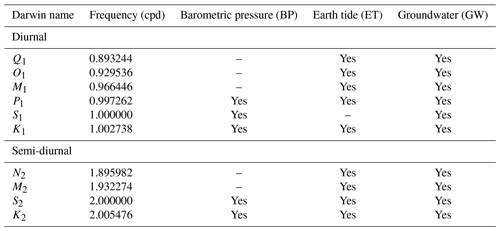analysed the groundwater response to both atmospheric pressure changes and Earth tides to quantify hydraulic and elastic properties. They reported that the frequency component S2 of the groundwater response exhibits a reliable and strong response to Earth tides but could not be used because it is contaminated by atmospheric pressure influences. For this reason, they concentrated their analysis on the Earth tide frequency M2. and used the groundwater response to atmospheric tides to estimate barometric efficiency, infer system confinement and calculate compressible storage. They used the tide frequency S2 in their work but did not take into account the effects of the phase lags between the Earth, atmospheric and groundwater tides that have been found to introduce errors in the analysis for higher levels of barometric efficiency.

published a method which objectively quantifies barometric efficiency (BE) using the groundwater response to atmospheric tides. Their approach considered the impact of phase lags between ${S}_{\mathrm{2}}^{\mathrm{AT}}$ and ${S}_{\mathrm{2}}^{\mathrm{ET}}$ in the amplitude response of the groundwater system. Their work demonstrated that (1) the harmonic addition theorem could be used to quantitatively disentangle the groundwater response to both Earth and atmospheric tides (EATs) acting at the same frequency, and (2) a theoretical Earth tide record is sufficient for this purpose. Because Earth tide records can be calculated with very high accuracy for any location on Earth and for time periods of general interest (e.g. McMillan et al.2019), this has opened the door for the widespread use of common barometric and groundwater pressure measurements (the latter in the form of standard well water levels) to characterise and quantify groundwater systems with little effort. compared different BE estimation methods and concluded that delivers robust results.

The method by is based on the assumption that the borehole water level is representative of subsurface pore pressure, i.e. there is an instantaneous and undamped response. Under such conditions, only the phase difference between the theoretical Earth and atmospheric tide drivers is required to correct the groundwater response amplitude. However, it has been established that the well water level response to Earth tide forces also depends on the hydraulic properties of the aquifer and well geometry and vadose zone air transport for conditions that are not confined . In fact, the amplitude and phase responses to Earth tides embedded in well water levels have been used to quantify subsurface hydraulic properties . reports an average phase shift of −12 between the M2 Earth tide potential and its well water level response. Consequently, an instantaneous and undamped groundwater response to Earth tides is not always a given and phase delays must be also considered when quantifying BE${}_{{S}_{\mathrm{2}}}$ from the groundwater response to atmospheric tides.

In this technical note, we generalise the method by by more completely disentangling the groundwater response to EATs in the frequency domain. We then illustrate the interpretative value of this new approach, using an example atmospheric pressure and borehole water level record that is strongly affected by EATs, followed by a verification of our results, using the well's barometric response function calculated in the time domain.

2 A generalised frequency domain method

## 2.1 Complete tidal disentanglement

Since the extension of the method published in to a generalised approach requires consideration of both the amplitudes and phases, we use complex numbers (denoted with a hat) for improved clarity. The complex numbers can be expressed with their real and imaginary components as follows:

$\begin{array}{}\text{(1)}& {\stackrel{\mathrm{^}}{z}}_{c}={a}_{c}+{b}_{c}i={A}_{c}{e}^{i{\mathrm{\Phi }}_{c}},\end{array}$

where ac and bc are the real and imaginary parts, respectively. $i=\sqrt{-\mathrm{1}}$, following the standard definition. The complex coefficients are related to harmonic amplitudes and phases as follows:

$\begin{array}{}\text{(2)}& {A}_{c}=\mathrm{abs}\left({\stackrel{\mathrm{^}}{z}}_{c}\right)=\sqrt{{a}_{c}^{\mathrm{2}}+{b}_{c}^{\mathrm{2}}},\end{array}$

and

$\begin{array}{}\text{(3)}& {\mathrm{\Phi }}_{c}=\mathrm{arg}\left({\stackrel{\mathrm{^}}{z}}_{c}\right)=\mathrm{arg}\left[\frac{{a}_{c}}{{b}_{c}}\right],\end{array}$

where the results within $-\mathit{\pi }\le {\mathrm{\Phi }}_{c}\le \mathit{\pi }$.

Throughout this paper, subscripts refer to the considered tidal components c, i.e. M2 (1.93227 cpd) and S2 (2 cpd). Superscripts stand for the measured parameter, i.e. GW stands for groundwater pressure head (measured as borehole water level and generally only required as a relative measurement), AT is atmospheric pressure (as water head equivalent) and ET is Earth tide (here, we use strain). Importantly, GW.ET and GW.AT represent the disentangled groundwater components response to Earth and atmospheric tides, respectively.

The method by can be generalised to allow a complete disentanglement of Earth and atmospheric tide influences from the groundwater response as follows:

1. The complex groundwater response to the Earth-tide-only driver for the M2 (1.93227 cpd) component is compared to the complex, theoretical Earth tide generated for the well geo-location, time and duration. Theoretical Earth tides can be calculated using software packages such as ETERNA (Wenzel1996), PyGTide (Rau2018) (which utilises the latest tidal catalogue), Baytap08 (Agnew2008) or TSoft (which was originally designed to analyse gravity records). Records for the theoretical Earth tide potential or gravity variations are highly accurate and avoid the need for measurements .

2. For some tidal components, for example S2 (2.0 cpd), the well water level responds to both Earth and atmospheric tides. The groundwater response magnitude to Earth tides only, for example at frequency M2 (1.93227 cpd), is assumed to be the same as for S2 because the frequencies are very close. Consequently, the S2 groundwater response to Earth tides alone can be calculated using the following:

$\begin{array}{}\text{(4)}& {\stackrel{\mathrm{^}}{z}}_{{S}_{\mathrm{2}}}^{\mathrm{GW}.\mathrm{ET}}=\frac{{\stackrel{\mathrm{^}}{z}}_{{M}_{\mathrm{2}}}^{\mathrm{GW}}}{{\stackrel{\mathrm{^}}{z}}_{{M}_{\mathrm{2}}}^{\mathrm{ET}}}{\stackrel{\mathrm{^}}{z}}_{{S}_{\mathrm{2}}}^{\mathrm{ET}}.\end{array}$
3. Since the measured well water level response for S2 contains a harmonic combination of both Earth and atmospheric tides, in the following :

$\begin{array}{}\text{(5)}& {\stackrel{\mathrm{^}}{z}}_{{S}_{\mathrm{2}}}^{\mathrm{GW}}={\stackrel{\mathrm{^}}{z}}_{{S}_{\mathrm{2}}}^{\mathrm{GW}.\mathrm{ET}}+{\stackrel{\mathrm{^}}{z}}_{{S}_{\mathrm{2}}}^{\mathrm{GW}.\mathrm{AT}},\end{array}$

the complex response to atmospheric tides alone can be calculated as follows:

$\begin{array}{}\text{(6)}& {\stackrel{\mathrm{^}}{z}}_{{S}_{\mathrm{2}}}^{\mathrm{GW}.\mathrm{AT}}={\stackrel{\mathrm{^}}{z}}_{{S}_{\mathrm{2}}}^{\mathrm{GW}}-{\stackrel{\mathrm{^}}{z}}_{{S}_{\mathrm{2}}}^{\mathrm{GW}.\mathrm{ET}}={\stackrel{\mathrm{^}}{z}}_{{S}_{\mathrm{2}}}^{\mathrm{GW}}-\frac{{\stackrel{\mathrm{^}}{z}}_{{M}_{\mathrm{2}}}^{\mathrm{GW}}}{{\stackrel{\mathrm{^}}{z}}_{{M}_{\mathrm{2}}}^{\mathrm{ET}}}{\stackrel{\mathrm{^}}{z}}_{{S}_{\mathrm{2}}}^{\mathrm{ET}}.\end{array}$

Unfortunately, Eq. (6) does not have a simplified expression consisting only of real valued numbers or functions.

It is important to note that our extended approach given in Eqs. (4)–(6) is correct irrespective of any processes that may affect the subsurface strain response to the stress induced by Earth tides. For example, delays may result from the physical characteristics of the aquifer borehole system but will be very similar for M2 and S2 because the frequencies are so close together. The theoretical Earth tide record merely helps to determine the absolute amplitudes and phases of the Earth tide response at S2 by accounting for the differences between the complex M2 and S2 determined from the theoretical Earth tide record to the absolute one at M2 measured in the well. The approach extends the method developed by because it considers all the signal phases in addition to their amplitudes. Since the inference of the well response to Earth tides is relative, the disentanglement can be done with any theoretical Earth tide signal, e.g. potentials, gravity variations or estimated strains.

## 2.2 Relationship between borehole water levels and subsurface pore pressure

assumed that the groundwater pressure head (i.e. the well water level) is representative of the subsurface pore pressure, i.e. an instantaneous and undamped response. However, calculation of the true BE based on subsurface pore pressure (outside of the well screen) requires a closer look at this relationship. Since tidal components comply well with harmonic functions, we can invoke the assumption that there is harmonically varying flow between the formation and the well (e.g.  Bredehoeft1967; Hsieh et al.1987; Rojstaczer1988). This groundwater flow problem has been solved analytically for confined and semi-confined (i.e. situations with vertical leakage through an overlying aquitard) conditions (see Appendix A). For the pressure relationship between subsurface and well water level, an amplitude ratio can be defined as follows:

$\begin{array}{}\text{(7)}& {A}_{c}^{r}=\mathrm{abs}\left[\frac{{\stackrel{\mathrm{^}}{z}}_{c}^{\mathrm{GW}}}{{\stackrel{\mathrm{^}}{z}}_{c}^{\mathrm{PP}}}\right]=\mathrm{abs}\left[\stackrel{\mathrm{^}}{H}\left({f}_{c},T,S,{r}_{\mathrm{wc}},{r}_{\mathrm{ws}}\right)\right].\end{array}$

Furthermore, a phase shift can be formulated as follows:

$\begin{array}{}\text{(8)}& \mathrm{\Delta }{\mathit{\varphi }}_{c}=\mathrm{arg}\left[\frac{{\stackrel{\mathrm{^}}{z}}_{c}^{\mathrm{GW}}}{{\stackrel{\mathrm{^}}{z}}_{c}^{\mathrm{PP}}}\right]=\mathrm{arg}\left[\stackrel{\mathrm{^}}{H}\left({f}_{c},T,S,{r}_{\mathrm{wc}},{r}_{\mathrm{ws}}\right)\right].\end{array}$

Here, the subscript c depicts any tidal component with distinct frequency. Superscripts GW and PP stand for well water level and subsurface pore pressure, respectively. The complex analytical function $\stackrel{\mathrm{^}}{H}$ is given in Appendix A and depends on the variables rwc and rws, which are the radius of the well casing and screen, respectively. b is the screen length. $T=K\cdot b$ and $S={S}_{\mathrm{s}}\cdot b$ are the transmissivity and storativity of the formation located along the well screen .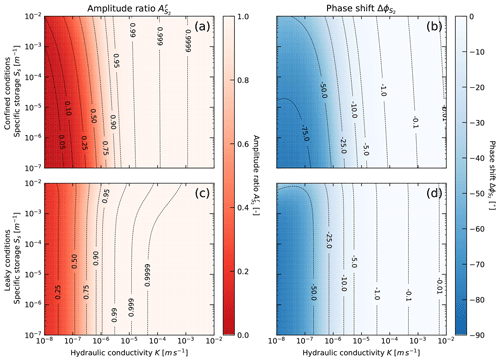Figure 1Amplitude ratio and phase shift relationship between subsurface pore pressure and well water level for harmonic forcing under fully confined conditions (a, b) and vertical water leakage under semi-confined conditions (c, d). The leaky aquitard has ${K}^{\prime }=\mathrm{5}×{\mathrm{10}}^{-\mathrm{5}}$ m/s and ${b}^{\prime }=\mathrm{2}$ m. The plots are calculated for a hypothetical well with radius of 25 mm and screen length of 1 m and realistic ranges of hydraulic conductivity and specific storage.

Figure 1 shows the amplitude ratio and phase shift calculated for the M2 Earth tide component (1.93227 cpd) and a hypothetical groundwater observation point with radius of 25 mm and screen length of 1 m. For the leaky aquifer solution, we assumed an aquitard with ${K}^{\prime }=\mathrm{5}×{\mathrm{10}}^{-\mathrm{5}}$ m/s and vertical thickness of 2 m. We used this value as a worst-case higher limit for an aquitard as the resulting amplitudes and phases provide a contrast to the confined case that is significant enough to visualise. The solutions illustrate that there is a frequency-dependent damping and phase shift in the well water level response to the aquifer pore pressure. Importantly, Fig. 1 highlights the following:

• The strongest modification of the harmonic response occurs for fully confined and not for leaky conditions (Fig. 1).

• both amplitude damping and phase shifts are mainly controlled by the subsurface hydraulic conductivity. For the confined case, ${A}_{{S}_{\mathrm{2}}}^{r}>\mathrm{0.99}$, which means that the relative error is smaller than 1 % for $K>\mathrm{1}×{\mathrm{10}}^{-\mathrm{5}}$ m/s and is therefore negligible. However, ${A}_{{S}_{\mathrm{2}}}^{r}$ dramatically decreases under lower hydraulic conductivity conditions and must therefore be considered for BEAT estimations.

• Ss does not significantly affect the well water level response, especially for $K>\mathrm{1}×{\mathrm{10}}^{-\mathrm{5}}$ m/s. However, the amplitude response to the Earth tide strain is proportional to Ss (Eq. 7).

Using Eqs. (4)–(7), a generalised method for objective BEAT quantification, using the groundwater response to atmospheric tides, for example at S2, can be formulated as follows:

$\begin{array}{}\text{(9)}& {\mathrm{BE}}_{{S}_{\mathrm{2}}}^{\mathrm{AT}}=\frac{\mathrm{1}}{{A}_{{S}_{\mathrm{2}}}^{r}}\mathrm{abs}\left[\frac{{\stackrel{\mathrm{^}}{z}}_{{S}_{\mathrm{2}}}^{\mathrm{GW}.\mathrm{AT}}}{{\stackrel{\mathrm{^}}{z}}_{{S}_{\mathrm{2}}}^{\mathrm{AT}}}\right].\end{array}$

Here, ${A}_{{S}_{\mathrm{2}}}^{r}$ accounts for the damping introduced by the subsurface well system under conditions of low hydraulic conductivity. Due to the closeness of the S2 and the M2 frequencies, we can assume that ${A}_{{S}_{\mathrm{2}}}^{r}\approx {A}_{{M}_{\mathrm{2}}}^{r}$.

The tidal disentanglement further enables estimation of the subsurface hydraulic conductivity (K) and specific storage (Ss), using the water level response to Earth tides. A negative phase shift between M2 and its groundwater response (well water level lags the Earth tide strain) requires horizontal flow in and out of the well and is therefore indicative of confined conditions . In this case, the amplitude and phase response of the well water level to an ET strain component is related as follows:

$\begin{array}{}\text{(10)}& {A}_{{M}_{\mathrm{2}}}^{e}=\mathrm{abs}\left[\frac{{\stackrel{\mathrm{^}}{z}}_{{M}_{\mathrm{2}}}^{\mathrm{GW}}}{{\stackrel{\mathrm{^}}{z}}_{{M}_{\mathrm{2}}}^{\mathrm{ET}}}\right]=\frac{{A}_{{M}_{\mathrm{2}}}^{r}}{{S}_{\mathrm{s}}},\end{array}$

(with aerial strain sensitivity equivalent to Eq. (A12) in Appendix A) and in the following:

$\begin{array}{}\text{(11)}& \mathrm{\Delta }{\mathit{\varphi }}_{{M}_{\mathrm{2}}}=\mathrm{arg}\left[\frac{{\stackrel{\mathrm{^}}{z}}_{{M}_{\mathrm{2}}}^{\mathrm{GW}}}{{\stackrel{\mathrm{^}}{z}}_{{M}_{\mathrm{2}}}^{\mathrm{ET}}}\right]\end{array}$

(which is equivalent to Eq. A13 in Appendix A). A positive phase shift is indicative of vertical water movement and semi-confined conditions . We note that the concept of BE describes a surface load sharing between matrix and pore water, which only exists under semi-confined to confined conditions, and that values of BE do not necessarily indicate the state of confinement .

## 2.3 Extraction of tidal components using harmonic least squares

The first step towards tidal disentanglement is to extract the tidal harmonics from the time series. Since the frequencies of the main tidal components are well known (e.g. McMillan et al.2019), a harmonic least squares (HALSs) estimation can be applied as follows (Agnew2007):

$\begin{array}{}\text{(12)}& \underset{{a}_{c},{b}_{c}}{min}\sum _{n=\mathrm{1}}^{N}{\left[{y}_{n}\left({t}_{n}\right)-\sum _{c=\mathrm{1}}^{C}\left[{a}_{c}\mathrm{cos}\left(\mathrm{2}\mathit{\pi }{f}_{c}{t}_{n}\right)+{b}_{c}\mathrm{sin}\left(\mathrm{2}\mathit{\pi }{f}_{c}{t}_{n}\right)\right]\right]}^{\mathrm{2}}.\end{array}$

Here, N is the number of discrete samples, yn(tn) is the sample value at time tn, and C is the total number of tidal components c with frequency fc. Table 1 shows the nine strongest tidal components that are generally observable in groundwater pressure measurements and are required for finding the best fit using Eq. (12). The coefficients ac and bc from Eq. (12) serve to derive the complex numbers representing each tidal constituent using Eq. (1). We further calculate the uncertainties of amplitudes and phases by propagating the covariances obtained from HALSs fitting (Eq. 12), using Eqs. (2) and (3). For details, refer to Appendix C.

Before extracting tidal harmonics, lower frequency variations should be removed. We suggest first applying a de-trending filter with a cut-off frequency of f<0.5 cpd. This improves the least squares approximation. It is important to note that the components used in the regression have to be customised for barometric pressure (BP), Earth tides (ET) and groundwater pressure head (GW) according to this table. For example, S1 is only contained in BP. This list is based on the findings by and .

3 Application

The three BEAT examples illustrated in show a limited impact of Earth tides relative to those of the atmospheric tides, resulting in a small magnitude correction at S2. To illustrate our new tidal disentanglement approach, we deliberately use a well water level record in which the Earth tide influence exceeds that of the atmospheric tides. This record originates from the well of BLM-1 in Death Valley (California, USA; WGS84; −116.471360 longitude, 36.408130 latitude; height – 688 m; casing and screen radius – 0.127 m; screen length – 106 m), which provided data for a previous analysis . The data set used here was recorded in the same well but at a later time. The data set was sampled at 15 min intervals (96 samples per day).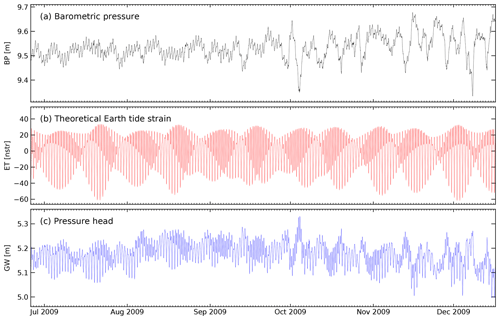Figure 2Time series of (a) barometric pressure (BP). (b) Theoretical Earth tide strains (ET) were calculated for the same duration and sampling rate and the well's geo-location using PyGTide (Rau2018) and (c) well water levels (GW), as measured in the well BLM-1 in Death Valley (California, USA). The vertical axes for BP and GW are limited to the same range for a visual comparison.

Figure 2 shows the barometric pressure (BP; black line, converted to pressure head equivalent), groundwater pressure head (GW; blue line, as measured in the well) and Earth tide strains (ET; red line) for BLM-1 over a time period of almost 6 months (25 June to 16 December 2009). The Earth tide strains were calculated using the Python package PyGTide (Rau2018) for the same time period and sampling frequency as the pressure measurements. It is interesting to note that both Earth tide and atmospheric pressure signatures are clearly visible in the groundwater response. In fact, the impact of both atmospheric pressure and Earth tide strains on the borehole water level is obvious just by looking at the raw data set.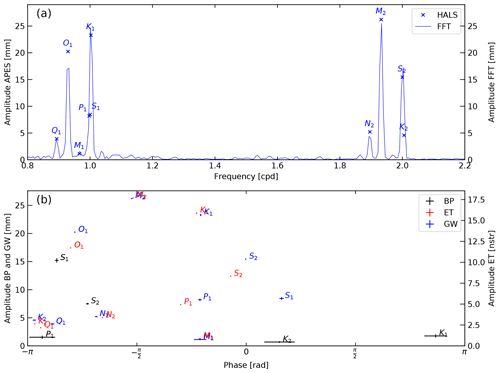Figure 3(a) A comparison of groundwater (GW) amplitudes for the interval $\mathrm{0.8}\le f\le \mathrm{2.2}$ cycle per day (cpd) derived using the general harmonic least squares estimation (Eq. 12) and the standard fast Fourier transform. (b) Amplitudes and phases of the most common tidal components in groundwater (GW), barometric pressure (BP) and Earth tides (ET) determined using harmonic least squares estimation. Note that horizontal and vertical error bars show the uncertainty of 1 standard deviation (Appendix C).

As a next step, we extracted the tidal harmonic components from all three time series (BP, ET and GW in Fig. 2) using the harmonic least squares estimation approach described in Sect. 2.3. Figure 3a shows the estimated amplitudes of the tidal components compared to a Fourier amplitude spectrum of the groundwater record. This example illustrates that separating tidal components with close-by frequencies is a clear challenge for the Fourier transform, even when the record duration is longer than that recommended by . It further highlights the well-known fact that spectral leakage can lead to errors in estimating the properties of the harmonic components (e.g. Tary et al.2014).

Figure 3b maps the amplitudes of the tidal components (Table 1) extracted from the original time series depicted in Fig. 2 against their phases. As expected, the strongest impact stems from the Earth-tide-only component M2. It is interesting to observe the similarity in the groundwater response magnitudes for all other Earth tide components, e.g. K1, O1, N2, Q1 and M1 (in decreasing order of impact). It is apparent that, at frequencies for which the groundwater record is influenced by both Earth and atmospheric tides, there is a substantial misalignment in amplitudes and phases of these components in groundwater in comparison to the forcing signals, e.g. see S2 or S1.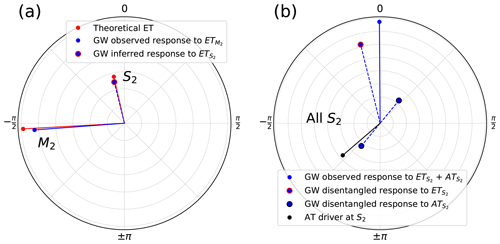Figure 4Polar plots showing amplitudes and phases derived from the fitting coefficients using Eqs. (1)–(3). (a) Results of the complex inference of the well response to Earth tides at S2 from the response at M2 (Eq. 4). (b) Harmonic disentanglement of the well response to atmospheric tides at S2 (Eq. 6). In (a), the Earth tide magnitude is scaled to improve comparison with the groundwater response.

To illustrate the tidal disentanglement, we use polar plots to visualise the key components. Figure 4a shows the amplitude and phase of the M2 component present in ET and GW. We apply the approach developed in Sect. 2.1 to infer the S2 response in the well that is caused by the Earth tides alone, using the theoretical Earth tide strains (note that the ET magnitude is scaled to improve comparison). Figure 4b shows the atmospheric tide driver at S2, the combined well water level response to EAT, the inferred groundwater response to S2 and the disentangled S2 response to atmospheric tides. All parameters and uncertainties calculated in this work are summarised in Table 2.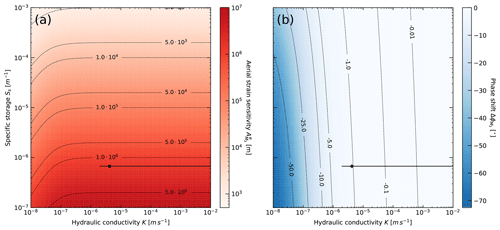Figure 5Well water level response to pore pressure for BLM-1 (well radius of 0.127 m and length of 106 m) and S2 frequency (2.0 cpd). Black dots represent the results for this well found by least squares fitting of the amplitude and phase response to the analytical solutions by . The horizontal and vertical (not visible) black lines depict the property ranges as a result of uncertainties in the aerial strain sensitivity and phase difference.

To account for the amplitude damping and phase shifting of the well in response to the harmonic pore pressure changes, we have used the dimensions of the well BLM-1 (see previous details) to calculate the solution space of the analytical solution for confined conditions (Appendix A) for the M2 frequency and for realistic limits of hydraulic conductivity ($\mathrm{1}×{\mathrm{10}}^{-\mathrm{8}} m/s) and specific storage ($\mathrm{1}×{\mathrm{10}}^{-\mathrm{7}}<{S}_{\mathrm{s}}<\mathrm{1}\cdot {\mathrm{10}}^{-\mathrm{3}}$ 1/m). The aerial strain sensitivity and phase shift between Earth tides and well response to M2 is shown in Fig. 5. We further used Eqs. (10) and (11) to estimate the hydraulic conductivity as $K\approx \mathrm{4.2}×{\mathrm{10}}^{-\mathrm{6}}$ m/s (ranging from $\mathrm{2.0}×{\mathrm{10}}^{-\mathrm{6}}$ to impossibly high values) and specific storage as ${S}_{\mathrm{s}}\approx \mathrm{6.7}×{\mathrm{10}}^{-\mathrm{7}}$ 1/m (ranging from $\mathrm{6.69}×{\mathrm{10}}^{-\mathrm{7}}$ to $\mathrm{6.77}×{\mathrm{10}}^{-\mathrm{7}}$ 1/m), which is representative for the materials along the well screen from the groundwater response to Earth tides (note the black annotations in Fig. 5). The Ss falls within the poroelastic limits determined by .

The damping of the amplitude in the well in this case is only ${A}_{{M}_{\mathrm{2}}}^{r}\approx \mathrm{0.998}$, which differs to the aquifer pore pressure by merely ≈0.2 %. It is important to note the high sensitivity to phase differences, i.e. small changes in $\mathrm{\Delta }{\mathit{\varphi }}_{{M}_{\mathrm{2}}}$ can cause large changes in the value of K (Eq. A13 and Fig. 5b). However, we note that as the sensitivity of the phase difference $\mathrm{\Delta }{\mathit{\varphi }}_{{M}_{\mathrm{2}}}$ to K drastically reduces in lower permeability settings (Fig. 1b), the confidence in K values will increase. Conveniently, this is also the value range where the amplitude response is most affected (Fig. 1a), allowing confidence in the robustness of our new BE${}_{{S}_{\mathrm{2}}}$ estimation approach for the investigated property ranges.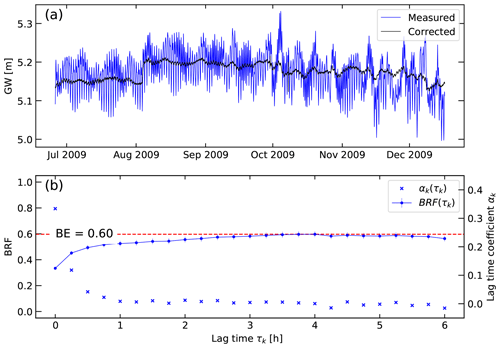Figure 6(a) Borehole pressure head (GW) measured and corrected for barometric and Earth tide influences. (b) Barometric response function (BRF) and BEBRF calculated using the records shown in Fig. 2 and the approach summarised in Appendix B.

Using Eq. (9), we calculate a BE${}_{{S}_{\mathrm{2}}}=\mathrm{0.60}$ from the disentangled groundwater response to atmospheric tides at S2 frequency. This is significantly different to the BE${}_{{S}_{\mathrm{2}}}=\mathrm{1.29}$ that results from using the method by . The latter is clearly erroneous, since it is larger than one, owing to the limited phase correction and leading to an incomplete disentanglement of the groundwater response to EATs. To verify our results, we independently calculated BEBRF for this location using the well-established barometric response function (BRF) approach developed and illustrated previously (a brief summary of the theory is given in Appendix BRasmussen and Crawford1997; Spane2002; Toll and Rasmussen2007; Butler et al.2011). The asymptotic value of the BRF at larger delay times represents confined conditions. The results further exhibit an exponential increase in the BRF over lag time (Fig. 6b). This is typical for water exchange controlled by the subsurface hydraulic conductivity where the shorter the lag time the stronger the deviation from BE , and it complies well with the frequency-dependent relationship shown in Fig. 1 .

The BRF-based BEBRF≈0.60 exactly matches that calculated using Eq. (9), confirming the robustness of the tidal disentanglement methodology we present in this work. The BRF is able to adequately remove the Earth and atmospheric influences on the measured groundwater levels (Fig. 6a). While BRFs are capable of indicating system confinement and estimating BE, they have not been used for estimating hydraulic properties.

Table 2Summary of the parameters, values and uncertainties calculated in this work.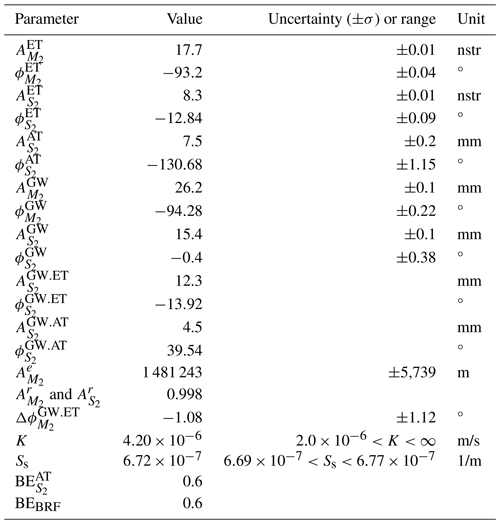The negative phase shift between Earth tides and groundwater pressure ($\mathrm{\Delta }{\mathit{\varphi }}_{{M}_{\mathrm{2}}}^{\mathrm{GW}.\mathrm{ET}}=-\mathrm{1.1}{}^{\circ }$; see Fig. 4a) can be interpreted as horizontal flow between the subsurface and the well, which occurs under confined conditions . Confined conditions can also be interpreted from the fact that the BRF time values reach a maximum value that is representative of the BE . Since this means that the well is screened in the confined zone, vertical loading due to atmospheric tides should also predominantly induce horizontal flow between the subsurface and the well with the same phase difference as given in response to Earth tides but considering the typical 180 (Fig. 4b) difference related to the subsurface stress balance . In fact, $\mathrm{\Delta }{\mathit{\varphi }}_{{S}_{\mathrm{2}}}^{\mathrm{GW}.\mathrm{AT}}=-\mathrm{9.8}{}^{\circ }$, which is also negative and very close to the phase delay in response to Earth tides.

Previous works have reported that a phase difference of 180 between the atmospheric tides and the groundwater response at S2 can be used to indicate confinement . However, this did not consider the fully disentangled groundwater response to atmospheric tides. Furthermore, has illustrated that the well response to barometric pressure depends on a number of processes accounting for the pressure propagation between surface and well, resulting in a frequency-dependent response of the borehole water level to barometric forcing. We propose that a combination of $\mathrm{\Delta }{\mathit{\varphi }}_{{M}_{\mathrm{2}}}$ and $\mathrm{\Delta }{\mathit{\varphi }}_{{S}_{\mathrm{2}}}$ could be diagnostic of the subsurface conditions at the borehole location. For example, a negative $\mathrm{\Delta }{\mathit{\varphi }}_{{M}_{\mathrm{2}}}$ is indicative of horizontal flow occurring under confined conditions for which $\mathrm{\Delta }{\mathit{\varphi }}_{{S}_{\mathrm{2}}}$ should equally be shifted to account for the 180 phase difference. A positive $\mathrm{\Delta }{\mathit{\varphi }}_{{M}_{\mathrm{2}}}$ has been attributed to vertical water movement under which the response of $\mathrm{\Delta }{\mathit{\varphi }}_{{S}_{\mathrm{2}}}$ could become diagnostic of the vertical pressure propagation and vadose zone properties . However, further research is required to develop a robust approach for detecting the confinement status at a particular frequency.

4 Conclusions

We present a frequency domain method to disentangle the groundwater response to Earth and atmospheric tides. It is a more general solution than that previously presented by since it is also applicable to subsurface environments with lower hydraulic conductivities, where measurable damping and time lags between formation pressure changes and well water level responses may be present. The approach only requires simultaneous records of barometric and groundwater pressure head in combination with theoretical Earth tide potential, gravity or strain variations, which are either standard measurements or can be calculated for any borehole geo-position using readily available software. Our novel approach exploits the fact that the complex harmonic components can be determined for each variable, i.e. barometric pressure, borehole water level and Earth tides from which subsurface flow direction (horizontal vs. vertical) in response to stresses allows the inference of confinement, estimation of barometric efficiency (BE), hydraulic conductivity and specific storage. We show that BE calculation using the borehole water level response to atmospheric tides may be substantially influenced by the hydraulic conductivity of the materials surrounding the well screen where $K<\mathrm{1}×{\mathrm{10}}^{-\mathrm{5}}$ m/s.

Our method enables an improved and rapid estimation of BE in general but especially for cases where the borehole water level is strongly influenced by Earth tides. Under such conditions, the influence of the phase difference between Earth tides and its groundwater response also has to be considered when revealing the atmospheric tide embedded in the S2 component of groundwater head measurements. The generalised solution further improves existing approaches and provides a next step towards a more reliable quantification of subsurface hydro-geomechanical properties using the groundwater response to Earth and atmospheric tides .

Appendix A: Well water level response to aquifer pore pressure

The following differential equation describes water flow in and out of a well under semi-confined (leaky) conditions:

$\begin{array}{}\text{(A1)}& \frac{{\mathit{\delta }}^{\mathrm{2}}s}{\mathit{\delta }{r}^{\mathrm{2}}}+\frac{\mathrm{1}\mathit{\delta }s}{r\mathit{\delta }r}-\frac{{K}^{\prime }s}{{b}^{\prime }Kb}=\frac{{S}_{\mathrm{s}}\mathit{\delta }s}{K\mathit{\delta }t}.\end{array}$

Here, s is the drawdown in the aquifer, r is the radius from the centre of the well, K and Ss are the hydraulic conductivity and specific storage of the aquifer, b is the aquifer thickness (here assumed to be the length of the well screen), and K and b are the hydraulic conductivity and thickness of the aquitard overlying the aquifer. has solved this equation for harmonically varying flow in and out of the well with the following boundary conditions:

$\begin{array}{}\text{(A2)}& s\left(\mathrm{\infty },t\right)=\mathrm{0},\end{array}$

and

$\begin{array}{}\text{(A3)}& \underset{r\to \mathrm{0}}{lim}\frac{r\mathit{\delta }s}{\mathit{\delta }r}=\frac{\mathit{\omega }{r}_{\mathrm{ws}}^{\mathrm{2}}\stackrel{\mathrm{^}}{x}}{\mathrm{2}Kb}\mathrm{sin}\left(\mathit{\omega }t\right).\end{array}$

The solution for the drawdown just outside the well screen rws is as follows:

$\begin{array}{}\text{(A4)}& {\stackrel{\mathrm{^}}{s}}_{w}={\stackrel{\mathrm{^}}{x}}_{\mathrm{0}}\cdot \stackrel{\mathrm{^}}{G},\end{array}$

and

$\begin{array}{}\text{(A5)}& \begin{array}{rl}\stackrel{\mathrm{^}}{G}& =\mathrm{0.5}i\cdot W\cdot {K}_{\mathrm{0}}\left[{\left[{W}^{\mathrm{2}}\left[{S}^{\mathrm{2}}+\frac{\mathrm{1}}{{q}^{\mathrm{2}}}\right]\right]}^{\mathrm{0.25}}\\ & \cdot \mathrm{exp}\left[\mathrm{0.5}i\left[\mathrm{atan}\left(qS\right)\right]\right]]\cdot \mathrm{exp}\left(i\mathit{\omega }t\right).\end{array}\end{array}$

In the following:

$\begin{array}{}\text{(A6)}& W=\frac{\mathit{\omega }{r}_{\mathrm{ws}}^{\mathrm{2}}}{Kb}\end{array}$

and

$\begin{array}{}\text{(A7)}& q=\frac{\mathit{\omega }{b}^{\prime }}{{K}^{\prime }}\end{array}$

and

$\begin{array}{}\text{(A8)}& \mathit{\omega }=\mathrm{2}\mathit{\pi }f.\end{array}$

Furthermore, K0 is the modified Bessel function of the second kind of order zero.

The fluctuating water level and the drawdown are related by the following:

$\begin{array}{}\text{(A9)}& \stackrel{\mathrm{^}}{x}={\stackrel{\mathrm{^}}{h}}_{f}-{\stackrel{\mathrm{^}}{s}}_{w},\end{array}$

where $\stackrel{\mathrm{^}}{x}$ is the borehole water level, $\stackrel{\mathrm{^}}{h}$ is the subsurface pressure head, and $\stackrel{\mathrm{^}}{s}$ is the drawdown. Equation (A4) can be substituted into Eq. (A9) to form a complex ratio of the well water level response to changing pore pressure as follows:

$\begin{array}{}\text{(A10)}& \stackrel{\mathrm{^}}{H}=\frac{\mathrm{1}}{\mathrm{1}+\stackrel{\mathrm{^}}{G}}.\end{array}$

For fully confined conditions, when ${K}^{\prime }\to \mathrm{0}$ and ${b}^{\prime }\to \mathrm{\infty }$, then the third term in Eq. (A1) becomes negligible, and the analytical solution becomes the same as that solved by . have shown that, in the following:

$\begin{array}{}\text{(A11)}& \stackrel{\mathrm{^}}{h}=\frac{\stackrel{\mathrm{^}}{\mathit{ϵ}}}{{S}_{\mathrm{s}}},\end{array}$

where ϵ is a strain. Using this relationship, the amplitude ratio and phase shift can be formulated as follows :

$\begin{array}{}\text{(A12)}& {A}_{r}=\mathrm{abs}\left[\frac{\stackrel{\mathrm{^}}{x}}{\stackrel{\mathrm{^}}{\mathit{ϵ}}}\right]{S}_{\mathrm{s}}=\frac{\mathrm{1}}{\sqrt{\left({E}^{\mathrm{2}}+{F}^{\mathrm{2}}\right)}},\end{array}$

and

$\begin{array}{}\text{(A13)}& \mathrm{\Delta }\mathit{\varphi }=\mathrm{arg}\left[\frac{\stackrel{\mathrm{^}}{x}}{\stackrel{\mathrm{^}}{\mathit{ϵ}}}\right]=-{\mathrm{tan}}^{-\mathrm{1}}\left[\frac{F}{E}\right].\end{array}$

In the following:

$\begin{array}{}\text{(A14)}& E=\mathrm{1}-\frac{\mathrm{2}\mathit{\pi }f{r}_{wc}^{\mathrm{2}}}{\mathrm{2}Kb}\left[\mathrm{\Psi }\mathrm{Ker}\left[{\mathit{\alpha }}_{w}\right]+\mathrm{\Phi }\mathrm{Kei}\left[{\mathit{\alpha }}_{w}\right]\right],\end{array}$

and

$\begin{array}{}\text{(A15)}& F=\frac{\mathrm{2}\mathit{\pi }f{r}_{\mathrm{wc}}^{\mathrm{2}}}{\mathrm{2}Kb}\left[\mathrm{\Phi }\mathrm{Ker}\left[{\mathit{\alpha }}_{w}\right]-\mathrm{\Psi }\mathrm{Kei}\left[{\mathit{\alpha }}_{w}\right]\right],\end{array}$

with

$\begin{array}{}\text{(A16)}& \mathrm{\Phi }=-\frac{{\mathrm{Ker}}_{\mathrm{1}}\left[{\mathit{\alpha }}_{w}\right]+{\mathrm{Kei}}_{\mathrm{1}}\left[{\mathit{\alpha }}_{w}\right]}{\sqrt{\mathrm{2}}{\mathit{\alpha }}_{w}\left({\mathrm{Ker}}_{\mathrm{1}}^{\mathrm{2}}\left[{\mathit{\alpha }}_{w}\right]+{\mathrm{Kei}}_{\mathrm{1}}^{\mathrm{2}}\left[{\mathit{\alpha }}_{w}\right]\right)},\end{array}$

and

$\begin{array}{}\text{(A17)}& \mathrm{\Psi }=-\frac{Ke{r}_{\mathrm{1}}\left[{\mathit{\alpha }}_{w}\right]-{\mathrm{Kei}}_{\mathrm{1}}\left[{\mathit{\alpha }}_{w}\right]}{\sqrt{\mathrm{2}}{\mathit{\alpha }}_{w}\left({\mathrm{Ker}}_{\mathrm{1}}^{\mathrm{2}}\left[{\mathit{\alpha }}_{w}\right]+{\mathrm{Kei}}_{\mathrm{1}}^{\mathrm{2}}\left[{\mathit{\alpha }}_{w}\right]\right)}.\end{array}$

Here, Ker and Kei are the kelvin functions of order zero, whereas Ker1 and Kei1 are the kelvin functions of order one. Finally, in the following:

$\begin{array}{}\text{(A18)}& {\mathit{\alpha }}_{w}=\sqrt{\frac{\mathit{\omega }{S}_{\mathrm{s}}b}{Kb}}{r}_{\mathrm{ws}}.\end{array}$
Appendix B: Calculating BE using the barometric response function

The well water level response to barometric pressure forcing in the time domain is referred to as the barometric response function (BRF). A BRF can be used to indicate confinement and estimate barometric efficiency . To account for time delays between changes in barometric pressure and their borehole water level changes, the differences are convoluted, and the time coefficients are determined by least squares regression. The method is as follows :

$\begin{array}{}\text{(B1)}& \underset{{\mathit{\alpha }}_{k},{\mathit{\beta }}_{k}}{min}\sum _{n=\mathrm{0}}^{N-\mathrm{1}}{\left[\mathrm{\Delta }\mathrm{GW}\left({t}_{n}\right)-\sum _{k=\mathrm{0}}^{K}\left[{\mathit{\alpha }}_{k}\mathrm{\Delta }\mathrm{BP}\left({t}_{n}-{\mathit{\tau }}_{k}\right)+{\mathit{\beta }}_{k}\mathrm{\Delta }\mathrm{ET}\left({t}_{n}-{\mathit{\tau }}_{k}\right)\right]\right]}^{\mathrm{2}},\end{array}$

where ΔGW, ΔBP and ΔET are the changes in borehole water level, barometric pressure, and theoretical Earth tides (potential, gravity or strain), respectively. tn is the time of sample n, where N is the total number of samples. K is the total number of time lags with relative time τk=kΔt. αk and βk are the time lag coefficients for the barometric and Earth tide response, respectively. Δt is the sampling period. It is a condition that KN. The time-based barometric response function for is calculated as follows:

$\begin{array}{}\text{(B2)}& \mathrm{BRF}\left({\mathit{\tau }}_{k}\right)=\sum _{k=\mathrm{0}}^{K}{\mathit{\alpha }}_{k}\left({\mathit{\tau }}_{k}\right).\end{array}$

According to , the BRF(tk) has a characteristic shape that is indicative of the system's confinement. If a system is confined, then BE can be calculated as follows :

$\begin{array}{}\text{(B3)}& \mathrm{BE}=max\left[\mathrm{BRF}\left({\mathit{\tau }}_{k}\right)\right].\end{array}$

This time-domain approach can also be used to remove barometric and Earth tide influences from the pressure head time series, for example, to reveal small responses to pumping that are otherwise buried in the natural signals. We note that we do not further interpret the Earth tide lag coefficients βk in this work.

Appendix C: Amplitude and phase uncertainty estimation

When HALSs optimisation (Eq. 12) is performed, a covariance matrix σ for the fitted coefficients ac and bc can be estimated. These can be propagated to obtain standard deviations for the amplitude (Eq. 2) as follows:

$\begin{array}{}\text{(C1)}& {\mathit{\sigma }}_{{A}_{c}}\approx \sqrt{{\left(\frac{{a}_{c}}{{A}_{c}}\right)}^{\mathrm{2}}{\mathit{\sigma }}_{{a}_{c}}^{\mathrm{2}}+{\left(\frac{{b}_{c}}{{A}_{c}}\right)}^{\mathrm{2}}{\mathit{\sigma }}_{{b}_{c}}^{\mathrm{2}}+\frac{\mathrm{2}{a}_{c}{b}_{c}}{{A}_{c}^{\mathrm{2}}}{\mathit{\sigma }}_{{a}_{c}{b}_{c}}},\end{array}$

and for the phase (Eq. 3) as follows:

$\begin{array}{}\text{(C2)}& {\mathit{\sigma }}_{{\mathit{\varphi }}_{c}}\approx \sqrt{{\left(\frac{{b}_{c}}{{a}_{c}^{\mathrm{2}}+{b}_{c}^{\mathrm{2}}}\right)}^{\mathrm{2}}{\mathit{\sigma }}_{{a}_{c}}^{\mathrm{2}}+{\left(\frac{-{a}_{c}}{{a}_{c}^{\mathrm{2}}+{b}_{c}^{\mathrm{2}}}\right)}^{\mathrm{2}}{\mathit{\sigma }}_{{b}_{c}}^{\mathrm{2}}-\frac{\mathrm{2}{a}_{c}{b}_{c}}{\left({a}_{c}^{\mathrm{2}}+{b}_{c}^{\mathrm{2}}{\right)}^{\mathrm{2}}}{\mathit{\sigma }}_{{a}_{c}{b}_{c}}}.\end{array}$

This further allows propagation to aerial strain sensitivity as follows:

$\begin{array}{}\text{(C3)}& {\mathit{\sigma }}_{{A}_{c}^{i,j}}\approx |{A}_{c}^{i,j}|\sqrt{{\left(\frac{{\mathit{\sigma }}_{{A}_{c}^{i}}}{{A}_{c}^{i}}\right)}^{\mathrm{2}}+{\left(\frac{{\mathit{\sigma }}_{{A}_{c}^{j}}}{{A}_{c}^{j}}\right)}^{\mathrm{2}}},\end{array}$

and the phase shift as follows:

$\begin{array}{}\text{(C4)}& {\mathit{\sigma }}_{\mathrm{\Delta }{\mathit{\varphi }}_{c}^{i,j}}\approx \sqrt{{\mathit{\sigma }}_{{\mathit{\varphi }}_{c}^{i}}^{\mathrm{2}}+{\mathit{\sigma }}_{{\mathit{\varphi }}_{c}^{j}}^{\mathrm{2}}}.\end{array}$

Here, the superscripts i and j stand for the two components that are related to each other, e.g. ET or GW.

Code and data availability

The code and data set are available on Figshare under https://doi.org/10.6084/m9.figshare.11316281 (Rau2020).

Author contributions

GCR conceived the idea for this work, analysed the data, made the figures and wrote the first draft. MOC contributed to method development through intensive technical discussions and reviews. RIA and PB improved this work by providing useful suggestions and edits.

Competing interests

The authors declare that they have no conflict of interest.

Acknowledgements

This technical note is based on a presentation given at the European Geosciences Union (EGU) General Assembly in the year 2020, which was reorganised as Sharing Geosciences Online due to the Covid-19 pandemic. We thank Paula Cutillo and Shannon Mazzei from the National Park Service (NPS) in California (USA) for providing the barometric and groundwater pressure data set. We acknowledge support from the KIT Publication Fund of the Karlsruhe Institute of Technology.

Financial support

This research has been supported by the European Commission Horizon 2020 Marie Skłodowska-Curie Actions (SubTideTools; grant no. 835852), an Independent Research Fellowship from the UK Natural Environment Research Council (grant no. NE/P017819/1), and the KIT Publication Fund of the Karlsruhe Institute of Technology. ​ ​ The article processing charges for this open-access ​ ​ ​ publication were covered by a Research ​ ​​ Centre of the Helmholtz Association.

Review statement

This paper was edited by Insa Neuweiler and reviewed by Todd Rasmussen and two anonymous referees.

References

Acworth, R. I. and Brain, T.: Calculation of barometric efficiency in shallow piezometers using water levels, atmospheric and earth tide data, Hydrogeol. J., 16, 1469–1481, https://doi.org/10.1007/s10040-008-0333-y, 2008. a

Acworth, R. I., Timms, W. A., Kelly, B. F., Mcgeeney, D. E., Ralph, T. J., Larkin, Z. T., and Rau, G. C.: Late Cenozoic paleovalley fill sequence from the Southern Liverpool Plains, New South Wales — implications for groundwater resource evaluation, Aust. J. Earth Sci., 62, 657–680, 2015. a

Acworth, R. I., Halloran, L. J. S., Rau, G. C., Cuthbert, M. O., and Bernardi, T. L.: An objective frequency domain method for quantifying confined aquifer compressible storage using Earth and atmospheric tides, Geophys. Res. Lett., 43, 611–671, https://doi.org/10.1002/2016GL071328, 2016. a, b, c, d, e, f, g, h, i, j, k, l, m, n

Acworth, R. I., Rau, G. C., Halloran, L. J. S., and Timms, W. A.: Vertical groundwater storage properties and changes in confinement determined using hydraulic head response to atmospheric tides, Water Resour. Res., 53, 2983–2997, https://doi.org/10.1002/2016WR020311, 2017. a

Agnew, D. C.: Baytap08: A Program for Analyzing Tidal Data, available at: https://igppweb.ucsd.edu/~agnew/Baytap/baytap.html (last access: 10 December 2020), 2008. a

Agnew, D. C.: 3.06 – Earth Tides, edited by: Schubert, G., Treatise on Geophysics, Elsevier, 163–195, https://doi.org/10.1016/B978-044452748-6.00056-0, 2007. a, b

Allègre, V., Brodsky, E. E., Xue, L., Nale, S. M., Parker, B. L., and Cherry, J. A.: Using earth-tide induced water pressure changes to measure in situ permeability: A comparison with long-term pumping tests, Water Resour. Res., 52, 3113–3126, https://doi.org/10.1002/2015WR017346, 2016. a, b, c, d

Bredehoeft, J. D.: Response of well-aquifer systems to Earth tides, J. Geophys. Res., 72, 3075–3087, https://doi.org/10.1029/JZ072i012p03075, 1967. a, b, c

Butler, J. J., Jin, W., Mohammed, G. A., and Reboulet, E. C.: New insights from well responses to fluctuations in barometric pressure, Ground Water, 49, 525–533, https://doi.org/10.1111/j.1745-6584.2010.00768.x, 2011. a, b

Clark, W. E.: Computing the barometric efficiency of a well, J. Hydraul. Div.-ASCE, 93, 93–98, http://cedb.asce.org/CEDBsearch/record.jsp?dockey=0014622 (last access: 10 December 2020), 1967. a

Cutillo, P. A. and Bredehoeft, J. D.: Estimating Aquifer Properties from the Water Level Response to Earth Tides, Ground Water, 49, 600–610, https://doi.org/10.1111/j.1745-6584.2010.00778.x, 2011. a, b

Davis, D. R. and Rasmussen, T. C.: A comparison of linear regression with Clark's Method for estimating barometric efficiency of confined aquifers, Water Resour. Res., 29, 1849–1854, https://doi.org/10.1029/93WR00560, 1993. a

Gieske, A. and De Vries, J. J.: An analysis of earth-tide-induced groundwater flow in eastern Botswana, J. Hydrol., 82, 211–232, https://doi.org/10.1016/0022-1694(85)90018-6, 1985. a

Hanson, J. M. and Owen, L. B.: Fracture Orientation Analysis by the Solid Earth Tidal Strain Method, Society of Petroleum Engineers, SPE Annual Technical Conference and Exhibition, 26-29 September, New Orleans, Louisiana, https://doi.org/10.2118/11070-MS, 1982. a

Hsieh, P. A., Bredehoeft, J. D., and Farr, J. M.: Determination of aquifer transmissivity from Earth tide analysis, Water Resour. Res., 23, 1824–1832, https://doi.org/10.1029/WR023i010p01824, 1987. a, b, c, d, e, f, g, h

Hsieh, P. A., Bredehoeft, J. D., and Rojstaczer, S. A.: Response of well aquifer systems to Earth tides: Problem revisited, Water Resour. Res., 24, 468–472, https://doi.org/10.1029/WR024i003p00468, 1988. a

McMillan, T. C., Rau, G. C., Timms, W. A., and Andersen, M. S.: Utilizing the Impact of Earth and Atmospheric Tides on Groundwater Systems: A Review Reveals the Future Potential, Rev. Geophys., 57, 281–315, https://doi.org/10.1029/2018RG000630, 2019. a, b, c, d, e, f, g, h, i, j

Merritt, M. L.: Estimating hydraulic properties of the Floridan Aquifer System by analysis of earth-tide, ocean-tide, and barometric effects, Collier and Hendry Counties, Florida, Tech. rep., https://doi.org/10.3133/wri034267, 2004. a, b, c, d

Rasmussen, T. C. and Crawford, L. A.: Identifying and Removing Barometric Pressure Effects in Confined and Unconfined Aquifers, Ground Water, 35, 502–511, https://doi.org/10.1111/j.1745-6584.1997.tb00111.x, 1997. a, b, c, d, e, f, g

Rau, G. C.: PyGTide: A Python module and wrapper for ETERNA PREDICT to compute synthetic model tides on Earth, Zenodo, https://doi.org/10.5281/zenodo.1346260, 2018. a, b, c

Rau, G. C.: Technical Note: Disentangling the groundwater response to Earth and atmospheric tides to improve subsurface characterisation, https://doi.org/10.6084/m9.figshare.11316281, 2020. a

Rau, G. C., Acworth, R. I., Halloran, L. J. S., Timms, W. A., and Cuthbert, M. O.: Quantifying Compressible Groundwater Storage by Combining Cross-Hole Seismic Surveys and Head Response to Atmospheric Tides, J. Geophys. Res.-Earth, 123, 1910–1930, https://doi.org/10.1029/2018JF004660, 2018. a

Ritzi, R. W., Sorooshian, S., and Hsieh, P. A.: The estimation of fluid flow properties from the response of water levels in wells to the combined atmospheric and Earth tide forces, Water Resour. Res., 27, 883–893, https://doi.org/10.1029/91WR00070, 1991. a

Roeloffs, E. A., Burford, S. S., Riley, F. S., and Records, A. W.: Hydrologic effects on water level changes associated with episodic fault creep near Parkfield, California, J. Geophys. Res., 94, 12387, https://doi.org/10.1029/jb094ib09p12387, 1989. a, b, c, d

Rojstaczer, S.: Determination of fluid flow properties from the response of water levels in wells to atmospheric loading, Water Resour. Res., 24, 1927–1938, https://doi.org/10.1029/WR024i011p01927, 1988. a, b, c, d, e, f, g, h

Rojstaczer, S. and Agnew, D. C.: The influence of formation material properties on the response of water levels in wells to Earth tides and atmospheric loading, J. Geophys. Res., 94, 12403, https://doi.org/10.1029/JB094iB09p12403, 1989.  a, b

Spane, F. A.: Considering barometric pressure in groundwater flow investigations, Water Resour. Res., 38, 14–1, https://doi.org/10.1029/2001WR000701, 2002. a, b

Tary, J. B., Herrera, R. H., Han, J., and Van Der Baan, M.: Spectral estimation - What is new? What is next?, Rev. Geophys., 52, 723–749, https://doi.org/10.1002/2014RG000461, 2014. a

Toll, N. J. and Rasmussen, T. C.: Removal of Barometric Pressure Effects and Earth Tides from Observed Water Levels, Ground Water, 45, 101–105, https://doi.org/10.1111/j.1745-6584.2006.00254.x, 2007. a

Turnadge, C., Crosbie, R. S., Barron, O., and Rau, G. C.: Comparing Methods of Barometric Efficiency Characterization for Specific Storage Estimation, Groundwater, 57, 844–859, https://doi.org/10.1111/gwat.12923, 2019. a, b

Van Camp, M. and Vauterin, P.: Tsoft: graphical and interactive software for the analysis of time series and Earth tides, Comput. Geosci., 31, 631–640, https://doi.org/10.1016/j.cageo.2004.11.015, 2005. a

van der Kamp, G. and Gale, J. E.: Theory of earth tide and barometric effects in porous formations with compressible grains, Water Resour. Res., 19, 538–544, https://doi.org/10.1029/WR019i002p00538, 1983. a

Wenzel, H.-G.: The nanogal software: Earth tide data processing package ETERNA 3.30, Bulletin d'Informations Mareés Terrestres, 124, 9425–9439, 1996. a

Xue, L., Brodsky, E. E., Erskine, J., Fulton, P. M., and Carter, R.: A permeability and compliance contrast measured hydrogeologically on the San Andreas Fault, Geochem. Geophy. Geosy., 17, 858–871, https://doi.org/10.1002/2015GC006167, 2016. a, b, c, d, e, f, g, h, i